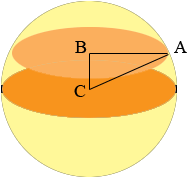SEARCH HOMEMath Central Quandaries & QueriesQuestion from Ed: I am writing a science fiction novel that involves a spherical space station with a radius of 800 meters. Inside, artificial gravity allows parallel floors set 4 meters apart. If you count the floor that has a radius of 800 meters as Floor 0, then the next floor up (Floor +1) would by 4 meters above the surface of Floor 0. There would then be Floor -1 4 meters down from Floor 0. This would continue until you reach the top or bottom floor, where there is at least 4 meters but less than 8 meters to the top or bottom of the sphere. Obviously the top and bottom floors would have the (same) smallest area, while Floor 0 would have about 2 million square feet. My problem is figuring out the total area of all of the floors, or for that matter, any particular floors or the total number of floors (the total of all the + floors, the - floors (these numbers will be the same) plus Floor 0. I tried using trigonometry couldn't derive the radius of any floor but Floor 0. Unfortunately I never took calculus, so if it is required I am really SOL. I would like the answers (total surface area of all floors and the number of floors). However, I would like to find a way to figure out how big the area of any specific floor is I also will need to make some floors not 4 meters apart but another amount, to accommodate tall equipment such as the fusion reactor. Thus if this reactor is the height of 14 meters, I'd have to know how to say, for example, that specific floors don't exist; e.g., floors +6 has the base of the reactor and floors +7, +8 and +9 are missing (and their area would have to be deducted from the grand total of all floors. Thanks. EdHi Ed,

In my diagram C is the centre of the sphere, B is the centre of some floor above C and A is a point on the circumference of this floor.CA is a radius of the sphere so its length is 800 metres, the length of BC is the distance between the floors and AB is a radius of the floor. Once you know the length of AB you can find the floor area.

ABC is a right triangle so Pythagoras theorem gives us

|AB|2 + |BC|2 = |CA|2.

Hence

|AB| = √(8002 - |BC|2) metres.

Good luck with the novel,
PennyMath Central is supported by the University of Regina and The Pacific Institute for the Mathematical Sciences.